true
true

Ramandeep K.

Sector-20, Chandigarh, India - 160020# Ramandeep K.

## Aspiring Science and Mathematics Teacher

Sector-20, Chandigarh, India - 160020.

1 Student taught

5.0

UrbanPro Rating

Details verified of Ramandeep K.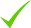IdentityEducation

Know how UrbanPro verifies Tutor details

Identity is verified based on matching the details uploaded by the Tutor with government databases.

## Overview

I am a dependable and reliable home tutor with one year of teaching multitude of high school subjects including Physics, Chemistry and Mathematics across CBSE board. Energetic and innovative individual with an innate ability to bring lesson plans to life from beyond the pages of textbooks and to devote my time to promote the importance of the science to today's youth. I participated in training for 6 months in a Government School in Rajasthan as a part of internship for Bachelor of Education.

## Languages Spoken

Punjabi Mother Tongue (Native)

English Proficient

Hindi Proficient

## Education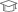Panjab University, Chandigarh 2015

Bachelor of Science (B.Sc.)Guru Nanam Dev University, Amritsar 2017

M.Sc PhysicsMaharaja Ganga Singh University, Bikaner, Rajasthan 2019

Bachelor of Education (B.Ed.)

Sector-20, Chandigarh, India - 160020

## Verified Info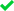Phone VerifiedEmail Verified

Report this Profile

Is this listing inaccurate or duplicate? Any other problem?

Type the letters as shown below *

Please enter the letters as show below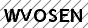Class 12 Tuition

Class LocationStudent's Home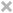Tutor's HomeOnline (video chat via skype, google hangout etc)

Years of Experience in Class 12 Tuition

1

Board

CBSE

CBSE Subjects taught

Chemistry, Physics, Mathematics

Taught in School or College

No

## Reviews (1)

this is test message this is test message this is test message this is test message this is test message this is test message this is test message

5.0 out of 5.0 1 review

Ramandeep K. https://s3-ap-southeast-1.amazonaws.com/tv-prod/member/photo/6571380-small.jpg Sector-20
5.0051
Ramandeep K.
J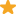"You are very good and calm teacher and I like how you explain every topic. Now, my basic concept are clear regarding physics thanks to you. "

Have you attended any class with Ramandeep? Write a Review

## FAQs

1. Which school boards of Class 12 do you teach for?

CBSE

2. Have you ever taught in any School or College?

No

3. Which classes do you teach?

I teach Class 10 Tuition, Class 11 Tuition and Class 12 Tuition Classes.

4. Do you provide a demo class?

Yes, I provide a free demo class.

5. How many years of experience do you have?

I have been teaching for 1 year.

If x^2+y^2+z^2 = xy+yz+zx This implies x^2+y^2+z^2-xy-yz-zx = 0 Put this value in standard formula x^3+y^3+z^3-3xyz = (x+y+z)(x^2+y^2+z^2-xy-yz-zx) ? x^3+y^3+z^3-3xyz = (x+y+z)(0) ? x^3+y^3+z^3-3xyz = 0 x^3+y^3+z^3 = 3xyz ...more

If x^2+y^2+z^2 = xy+yz+zx

This implies x^2+y^2+z^2-xy-yz-zx = 0

Put this value in standard formula

x^3+y^3+z^3-3xyz = (x+y+z)(x^2+y^2+z^2-xy-yz-zx)

? x^3+y^3+z^3-3xyz = (x+y+z)(0)

? x^3+y^3+z^3-3xyz = 0

x^3+y^3+z^3 = 3xyz

Dislike Bookmark
Class 12 Tuition 5.0

Class LocationStudent's HomeTutor's HomeOnline (video chat via skype, google hangout etc)

Years of Experience in Class 12 Tuition

1

Board

CBSE

CBSE Subjects taught

Chemistry, Physics, Mathematics

Taught in School or College

No

Class 11 Tuition 5.0

Class LocationStudent's HomeTutor's HomeOnline (video chat via skype, google hangout etc)

Years of Experience in Class 11 Tuition

1

Board

CBSE, State

Taught in School or College

No

State Syllabus Subjects taught

Mathematics, Physics, Chemistry

Class 10 Tuition 4.5

Class LocationStudent's HomeTutor's HomeOnline (video chat via skype, google hangout etc)

Years of Experience in Class 10 Tuition

1

Board

State, CBSE

CBSE Subjects taught

Mathematics, Science, English

Taught in School or College

No

State Syllabus Subjects taught

English, Mathematics, Science

this is test message this is test message this is test message this is test message this is test message this is test message this is test message

5.0 out of 5.0 1 review

Ramandeep K.
J"You are very good and calm teacher and I like how you explain every topic. Now, my basic concept are clear regarding physics thanks to you. "

Have you attended any class with Ramandeep? Write a Review

If x^2+y^2+z^2 = xy+yz+zx This implies x^2+y^2+z^2-xy-yz-zx = 0 Put this value in standard formula x^3+y^3+z^3-3xyz = (x+y+z)(x^2+y^2+z^2-xy-yz-zx) ? x^3+y^3+z^3-3xyz = (x+y+z)(0) ? x^3+y^3+z^3-3xyz = 0 x^3+y^3+z^3 = 3xyz ...more

If x^2+y^2+z^2 = xy+yz+zx

This implies x^2+y^2+z^2-xy-yz-zx = 0

Put this value in standard formula

x^3+y^3+z^3-3xyz = (x+y+z)(x^2+y^2+z^2-xy-yz-zx)

? x^3+y^3+z^3-3xyz = (x+y+z)(0)

? x^3+y^3+z^3-3xyz = 0

x^3+y^3+z^3 = 3xyz

Dislike Bookmark

Ramandeep K. describes herself as Aspiring Science and Mathematics Teacher. She conducts classes in Class 10 Tuition, Class 11 Tuition and Class 12 Tuition. Ramandeep is located in Sector-20, Chandigarh. Ramandeep takes at students Home. She has 1 years of teaching experience . Ramandeep has completed Bachelor of Science (B.Sc.) from Panjab University, Chandigarh in 2015, M.Sc Physics from Guru Nanam Dev University, Amritsar in 2017 and Bachelor of Education (B.Ed.) from Maharaja Ganga Singh University, Bikaner, Rajasthan in 2019. She is well versed in Punjabi, English and Hindi. Ramandeep has got 1 reviews till now with 100% positive feedback.

•• Want to learn from Ramandeep?

• Contact Now
X

Recommended Profiles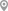Sector-20, ChandigarhSector-20, ChandigarhSector-20, ChandigarhSector-20, ChandigarhSector-20, ChandigarhSector-20, Chandigarh

X

Let us shortlist and give the best tutors and institutes.

or

Send Enquiry to Ramandeep

Let Ramandeep know you are interested in their class